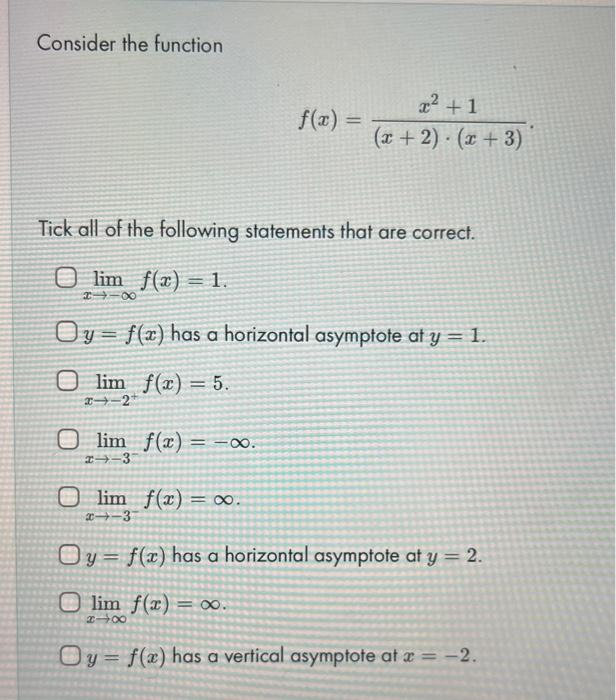Home / Expert Answers / Calculus / consider-the-function-f-x-frac-x-2-1-x-2-cdot-x-3-tick-all-of-the-following-stateme-pa758

# (Solved): Consider the function $f(x)=\frac{x^{2}+1}{(x+2) \cdot(x+3)}$ Tick all of the following stateme ...Consider the function $f(x)=\frac{x^{2}+1}{(x+2) \cdot(x+3)}$ Tick all of the following statements that are correct. $\lim _{x \rightarrow-\infty} f(x)=1 \text {. }$ $$y=f(x)$$ has a horizontal asymptote at $$y=1$$. $\begin{array}{l} \lim _{x \rightarrow-2^{+}} f(x)=5 . \\ \lim _{x \rightarrow-3^{-}} f(x)=-\infty . \\ \lim _{x \rightarrow-3^{-}} f(x)=\infty . \end{array}$ $$y=f(x)$$ has a horizontal asymptote at $$y=2$$. $\lim _{x \rightarrow \infty} f(x)=\infty$ $$y=f(x)$$ has a vertical asymptote at $$x=-2$$.

We have an Answer from Expert